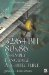Boolean Operations upon Floating-Point NumbersBoolean Operations upon Floating-Point Numbers

For the SSE there is no mixing and matching of types; however, any masking value can be used provided that each element mask is also a valid number. A full masking value of 0 versus 1 is allowed since they are valid numbers. 0 is zero and 1 (all bits set) is a QNaN floating-point value. So if ANDed with a floating-point value the value will either keep existing or become zero. A value with only the MSB set is also valid, such as 80000000h as (0, 0) is a negative floating-point zero. But one should be careful as this could be used to complement the sign bit (A = 0.0 A) and thus negate a number. A = A, when used in an XOR operation.

There is an exception to this and that is the use of 3DNow! With this instruction set integers and floating-point values can be mixed and matched.

A quick preview of floating-point numbers illuminates the individual bits and their associations with their particular components .

By manipulating key bits the number can be affected. For example, for a 32-bit single-precision floating-point number the sign bit is bit #31 080000000h. So setting it (1) indicates the number is negative, and resetting it (0) indicates the number is positive. Other bit manipulations can be conceived by re-examining the bits described at the beginning of this chapter.

This coincidently happens to be an example of branchless code. It uses bit blending using masks generated by the results of the floating-point comparison 0 : 1.

a = (a   b) ? c : d;
 1 2 3 4 5 6 7 = > ~ORD < ORD
cmpps   xmm0, xmm1,   5   ; (a     b) ? 1 : 0 movaps  xmm7, xmm0        ; Copy the bit mask andps   xmm0, xmm2        ; a = a   c andnps  xmm7, xmm3        ; t =32/64-Bit 80x86 Assembly Language Architecture
ISBN: 1598220020
EAN: 2147483647
Year: 2003
Pages: 191

Similar book on Amazon